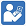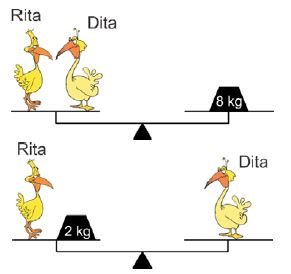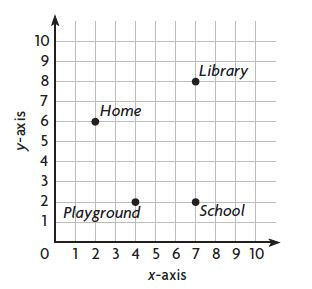Math - Grade 5

Year Published: 2015
Year Published: 2019

Math Books

Select from a variety of school and library books to prepare for the end of year common core upcoming math test.Recently Added Tests (see all... )

Grade 5 Homework Resolved
Year Published: 2015

Grade 5 Homework MyMath
Year Published: 2019

Grade 5 Homework MyMath
Year Published: 2019

Grade 5 Homework MyMath
Year Published: 2019

Grade 5 Homework Resolved - NEW
Year Published: 2016

More Tests

View hundreds more test and work sheets browseable by curriculum category.

What is Common Core

The Common Core is a set of high-quality academic standards in mathematics and English language arts/literacy (ELA).

These learning goals outline what a student should know and be able to do at the end of each grade.

What Parents Should Know

Today’s students are preparing to enter a world in which colleges and businesses are demanding more than ever before.

To ensure all students are ready for success after high school, the Common Core State Standards establish clear, consistent guidelines for what every student should know and be able to do in math and English language arts from kindergarten through 12th grade.

Common core - Common mistakes

Use the repeated-addition strategy to solve 5x3.

If you answer the question with 5+5+5=15, you would be wrong.

The correct answer is 3+3+3+3+3.

Mathematically, both are correct. But under Common Core, you're supposed to read 5x3 as "five groups of three." So "three groups of five" is wrong.

Getting started

Math Practice 101 gives you a powerful, patterns-based way to learn and practice for the upcoming Common Core Standard Test

Get more didactic materials

We offer school books, library books, test and practice worksheets to help you sharpen your math skills.

Free Online Tutor

As a registered member, you have access to our online tutors that will help you with any questions you might have.

Featured problem of the day

The two pictures of the balanced scales show the birds Rita and Dita.How much does Dita weigh?

• 2 kg
• 3 kg
• 4 kg
• 5 kg

Lesson CheckWhich ordered pair describes the location of the playground?

• $$\left(2,4\right)$$
• $$\left(4,2\right)$$
• $$\left(3,1\right)$$
• $$\left(1,3\right)$$
• $$(7\times60)\times(7\times3)$$
• $$(7+60)\times(7+3)$$
• $$(7\times60)+(7\times3)$$
• $$7+(60\times3)$$